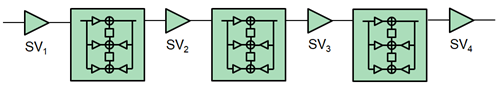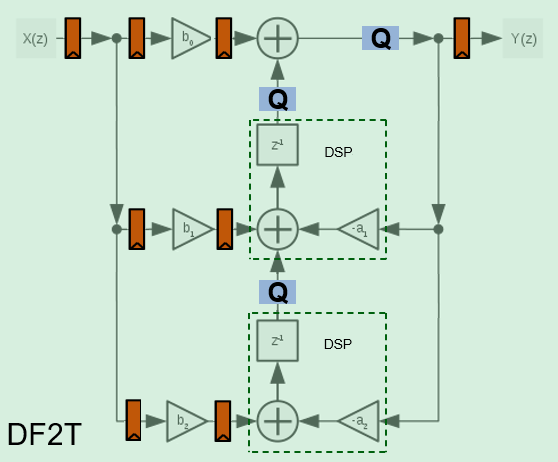• Library:
• DSP HDL Toolbox / Filtering

•## Description

A biquad filter is a form of infinite-impulse response (IIR) filter where the numerator and denominator are split into a series of second-order sections connected by gain blocks. This type of filter can replace a large FIR filter that uses an impractical amount of hardware resources. Designs often use biquad filters as DC blocking filters or to meet a specification originally implemented with an analog filter, such as a pre-emphasis filter.

## Ports

### Input

expand all

Input data, specified as a scalar or column vector of real values. When the input has an integer or fixed-point data type, the block uses fixed-point arithmetic for internal calculations.

Vector input is supported only when you set Filter structure to `Pipelined feedback form`. The block accepts vectors up to 64 samples, but large vector sizes can make the calculation of internal data types challenging. Vector sizes of up to 16 samples are practical for hardware implementation.

`double` and `single` data types are supported for simulation, but not for HDL code generation.

Data Types: `fixed point` | `single` | `double` | `int8` | `int16` | `int32` | `uint8` | `uint16` | `uint32`

Control signal that indicates if the input data is valid. When valid is `1` (`true`), the block captures the values from the input data port. When valid is `0` (`false`), the block ignores the values from the input data port.

Data Types: `Boolean`

### Output

expand all

Filtered output data, returned as a scalar or column vector of real values. The output dimensions match the input dimensions. When the input data type is a floating-point type, the output data inherits the data type of the input data. When the input data type is an integer type or a fixed-point type, the Output parameter on the Data Types tab controls the output data type.

Data Types: `fixed point` | `single` | `double` | `int8` | `int16` | `int32` | `uint8` | `uint16` | `uint32`

Control signal that indicates if the data from the output data port is valid. When valid is `1` (`true`), the block returns valid data from the output data port. When valid is `0` (`false`), the values from the output data port are not valid.

Data Types: `Boolean`

## Parameters

expand all

### Main

Both the `Direct form II` and ```Direct form II transposed``` architectures are pipelined and quantized to fit well into FPGA DSP blocks. The output of these filters matches the output of the DSP System Toolbox™ System objects `dsp.SOSFilter` and `dsp.FourthOrderSectionFilter`. These architectures minimize the number of multipliers used by the filter but have a critical path through the feedback loop and sometimes cannot achieve higher clock rates.

`Pipelined feedback form` implements a pipelined architecture that uses more multipliers than either direct-form II structure, but achieves higher clock rates after synthesis. Frame-based input is supported only when you use `Pipelined feedback form`. The output of the pipelined filter is slightly different than the DSP System Toolbox functions `dsp.SOSFilter` and `dsp.FourthOrderSectionFilter` because of the timing of data samples applied in the pipelined filter stages.

Specify the numerator coefficients as a matrix of NumSections-by-3 values. NumSections is the number of second-order filter sections. The block infers the number of filter sections from the size of the numerator and denominator coefficients. The numerator coefficient and denominator coefficient matrices must be the same size. The default filter has one section.

Specify the denominator coefficients as a matrix of NumSections-by-3 values. The block assumes the first denominator coefficient of each section is `1.0`. NumSections is the number of second-order filter sections. The block infers the number of sections from the size of the numerator and denominator coefficients. The numerator coefficient and denominator coefficient matrices must be the same size. The default filter has one section.

Specify the gain values as a vector of up to NumSections+1 values. NumSections is the number of second-order filter sections. The block infers the number of sections from the size of the numerator and denominator coefficients. If the vector has only one value, the block applies that gain before the first section. If you specify fewer values than there are filter sections, the block sets the remaining section gain values to one. The diagram shows a 3-section filter and the locations of the four scale values before and after the sections.Implementing these gain factors outside the filter sections reduces the multipliers needed to implement the numerator of the filter.

### Data Types

Rounding mode for type-casting the output and accumulator values to the data types specified by the Output and Accumulator parameters. When the input data type is floating point, the block ignores this parameter. For more details, see Rounding Modes.

Overflow handling for type-casting the output and accumulator values to the data types specified by the Output and Accumulator parameters. When the input data type is floating point, the block ignores this parameter. For more details, see Overflow Handling.

The block casts the numerator coefficients to this data type. The quantization rounds to the nearest representable value and saturates on overflow. When the input data type is floating point, the block ignores this parameter.

The block returns a warning if the data type of the coefficients does not have enough fractional length to represent the coefficients accurately.

The block casts the denominator coefficients to this data type. The quantization rounds to the nearest representable value and saturates on overflow. When the input data type is floating point, the block ignores this parameter.

The block returns a warning if the data type of the coefficients does not have enough fractional length to represent the coefficients accurately.

The block casts the scale values to this data type. The quantization rounds to the nearest representable value and saturates on overflow. When the input data type is floating point, the block ignores this parameter.

The block casts the internal accumulator signals (as indicated in the diagrams in the Algorithms section) to this data type. The quantization uses the settings of the Rounding mode and Saturate on integer overflow parameters. When the input data type is floating point, the block ignores this parameter.

The block casts the output of the filter to this data type. The quantization uses the settings of the Rounding mode and Saturate on integer overflow parameters. When the input data type is floating point, the block ignores this parameter.

## Algorithms

expand all

The direct form II and direct form II transposed architectures are pipelined and quantized to fit well into FPGA DSP blocks. The architecture diagrams show the accumulator quantization points and pipeline registers for the direct form II and direct form II transposed architectures. The dashed lines indicate how the multiply-add operation fits into a DSP block on an FPGA.The transposed biquad filter implementations have a long critical path through the feedback loop between poles z-1 and z-2. They use the lowest number of multipliers but are not suitable for high-speed applications.

The pipelined biquad architecture uses more multipliers but reduces the critical path and achieves higher clock rates. The filter uses the Parhi method , which adds pipeline registers by increasing the order of the denominator, and then compensates for the modified denominator by adding poles in the numerator. The pipelined denominator uses order z-4 and z-8 rather than z-1 and z-2. Since the poles are less than one for a stable filter, the poles from the higher orders are smaller than the starting values, which adds to filter stability.

To support frame-based input, the pipelined architecture increases the order of the denominator to the power of the input size. A frame-based pipelined denominator uses z-N*4 and z-N*8, where N is the size of the input vector. The new numerator that compensates for the additional poles in the denominator has `2*N*4-1` coefficients. This implementation uses more resources but supports high-throughput applications.

 Chung, Jin-Gyun, and Keshab K. Parhi. Pipelined Lattice and Wave Digital Recursive Filters. Boston: Springer US, 1996. https://doi.org/10.1007/978-1-4613-1307-6.